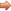# JavaScript & jQuery Tutorials

JS HomeJS ReferenceComparison

## Comparison operators

Used when we want to compare operands.

### Description

Comparison operators allow us to compare operands in various ways.

Operands can be numerical or string values and are compared by standard lexicographical ordering using Unicode values.

`null` and `undefined` types are equal but not strictly equal.

## Type Converting Comparators

These comparators attempt to convert the operands for comparison.

`==` Equal

`!=` Not equal

`<` Less than

`<=` Less than or equal

`>` Greater than

`>=` Greater than or equal

### Type Converting Rules

`null` and `undefined` types are equal.

#### Number and String Comparison

The string is converted to a number value and then JavaScript attempts to convert the string numeric literal to a `Number` type as follows:

1. A mathematical value is derived from the string numeric literal.
2. The value is rounded to the nearest `Number` type value.

#### Boolean Comparison

For boolean conversion the following happens:

• If the boolean value is `true` it is converted to the number 1
• If the boolean value is `false` it is converted to the number +0

#### Object Comparison

For object conversion where both operands are objects no conversion takes place. The equality test is true only if both objects refer to the same object, otherwise object conversion applies as follows:

• JavaScript attempts to return the default value for the object when compared with a number or string.
• The `toString` and `valueOf` are used to convert the object to a primitive value, a `Number` or `String` value. If this attempt to convert the object fails, a runtime error is generated.

### Equal comparator `==`

The equal comparator returns `true` if the operands are equal and does the following before comparing the two operands.

For operands of different types, JavaScript converts the operands then applies strict comparison via the === operator in the following order.

1. Where either operand is a number or boolean, both operands are converted to numbers when possible.
2. Where either operand is a string, the other operand is converted to a string when possible.
3. When both operands are JavaScript objects , they are equal when both equate to the same memory reference.

### Examples

The code below does some equality comparison.

``````
//Create and compare some variables
var aVariable = 1, bVariable = '1', cVariable = true;

// The string value of bVariable converted to 1 so true
if (aVariable == bVariable) {
} else {
}
// The boolean value of cVariable converted to 1 so true}
if (aVariable == cVariable) {
} else {
}

// The boolean value of cVariable converted to 1 and
// then string value of bVariable converted to 1 so true}
if (bVariable == cVariable) {
} else {
}

``````

Press the button below to action the above code:

### Not Equal comparator `!=`

The not equal comparator returns `true` if the operands are NOT equal and does the following before comparing the two operands.

For operands of different types, JavaScript attempts to convert an appropriate comparison type. When both operands are JavaScript objects , they are not equal when both equate to different memory references.

### Examples

The code below does some inequality comparisons.

``````
//Create and compare some variables for inequality
var aVariable = 1, bVariable = '1', cVariable = true;

// The string value of bVariable converted to 1 so false
if (aVariable != bVariable) {
} else {
}

// The boolean value of cVariable converted to 1 so false
if (aVariable != cVariable) {
} else {
}

// The boolean value of cVariable converted to 1 and
// then string value of bVariable converted to 1 so false
if (bVariable != cVariable) {
} else {
}

``````

Press the button below to action the above code:

### Less than comparator `<`

Returns `true` if the left operand is less than the right operand.

### Less than or equal comparator `<=`

Returns `true` if the left operand is less than or equal to the right operand.

### Greater than comparator `>`

Returns `true` if the left operand is greater than the right operand.

### Greater than or equal comparator `>=`

Returns `true` if the left operand is greater than or equal to the right operand.

## Strict Comparators

These comparators must have the same type.

`===` Strict equal

`!==` Strict not equal

### Strict Comparison Rules

`null` and `undefined` types are not strictly equal.

#### Number Comparison

Numbers are strictly equal when they have the same numerical value.

• `NaN()` is not equal to anything, including `NaN`.
• Positive and negative zeros are equal.

#### Boolean Comparison

Booleans are strictly equal if both are `true` or `false`

#### String Comparison

Strings are strictly equal when they have the same sequence of characters, in the same positions with the same length.

#### Object Comparison

Objects are strictly equal if they refer to the same object.

## Related Tutorials

JavaScript Intermediate Tutorials - Lesson 3 - Conditional Statements